# Speed Distance And Time : Download Mathematics Study Notes Free PDF For REET ExamMathematics is an equally important section for REET, MPTET & DSSSB Exams and has even more abundant importance in some other exams conducted by central or state govt.

To let you make the most of Mathematics section, we are providing important facts related to the Speed Distance And Time. At least 1-2 questions are asked from this topic in most of the teaching exams. We wish you all the best of luck to come over the fear of the Mathematics section.

How to Overcome Exam Fever, Especially When You Fear Maths

## SPEED, DISTANCE AND TIME

SPEED

Distance covered per unit time is called speed.

For example, Speed = Distance / time or

Distance = Speed × Time or

Time = Distance/Speed

• If two bodies travel with the same speed, Distance covered a Time (Direct Variation).
• If two bodies travel for the same period of time, Distance covered a Speed (Direct Variation).
• If two bodies travel the same distance.

Distance is normally measured in kilometres, metres or miles; time in hours or seconds and speed in km/hr or kmph, miles/hr or mph and metres/second or m/s.

Conversion of units:

• kmph to m/sec = kmph× 5/18

Example: A man walking at the rate of 8 km/h crosses a bridge in 30 minutes. The length of the bridge (in metres) is: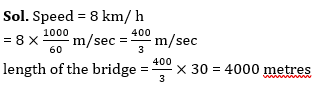• m/sec to kmph = m/sec ×18/5

Mathematics Study Notes For All Teaching Exams

Example: A Lady crosses a road 350 metres wide in 70 seconds. Her speed in km/h is: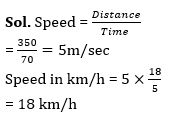In the case of moving trains, three different situations need to be considered. If the train is crossing a platform or a bridge, the distance covered by the train in the crossing is equal to the length of the train plus the length of the platform or bridge. If two trains pass each other or travelling in the same direction or in opposite directions, the total distance covered in the crossing or the overtaking, as the case may be is equal to the sum of the lengths of the two trains.

AVERAGE SPEED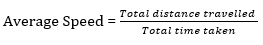AVERAGE SPEED of a moving body is NOT EQUAL to the AVERAGE OF THE SPEEDS.

If an object travels from point A to point B with a speed of p and back to point A (from point B) with a speed of q, then the average speed of the body can be calculated as 2pq/ (p + q). this does not depend on the distance between A and B.

If an object covers part of the journey at speed p and the remaining part of the journey at speed q and the distances of the two parts of the journey are in the ratio a:b, then the average speed for the entire journey is (a + b) pq /(aq + bp).

Get free Study material for REET Exam

Example: A travel for 5 hours at the 8 km/hour and for 4 hours at the 7 km/hours the average speed of the journey in km/hour is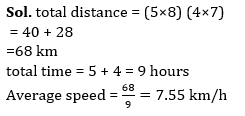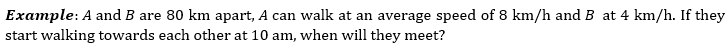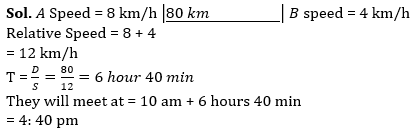RELATIVE SPEED

The speed of one body in relation to another moving body is called the relative speed of these two bodies, i.e., it is the speed of one moving body as observed, from the second moving body.

If two bodies are moving in the same direction, the relative speed is equal to the difference of the speeds of the two bodies.

If two bodies are moving in opposite directions, the relative speed is equal to the sum of the speeds of the two bodies.

Example: Find the time taken by a train of length 100 m running at a speed of 72 kmph to cross another train of length 200 m running at a speed of 63 kmph in the same direction.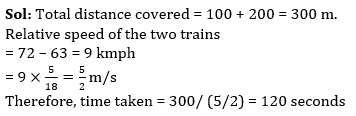Practice REET Subject wise Quiz

Example: Two trains are running 60 km/h and 30 km/h respectively in the same direction. The fast train completely passes a child sitting in the slower train in 6 seconds. the length of the faster train is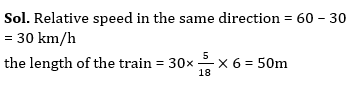BOATS AND STREAMS

Problems related to boats and streams are different in the computation of relative speed from those of trains/cars.

When a boat is moving in the same direction as the stream or water current, the boat is said to be moving WITH THE STREAM OR CURRENT.

When a boat is moving in a direction opposite to that of the stream or water current, it is said to be moving AGAINST THE STREAM OR CURRENT.

If the boat is moving with a certain speed in water that is not moving, the speed of the boat is then called the SPEED OF THE BOAT IN STILL WATER.

When the boat is moving upstream, the speed of the water opposes (and hence reduces) the speed of the boat.

When the boat is moving downstream, the speed of the water aids and adds to the speed of the boat. So, we have

 Speed of the boat against stream = Speed of the boat in still water – Speed of the stream Speed of the boat with the stream = Speed of the boat in still water + Speed of the stream

These two speeds, the speed of the boat against the stream and the speed of the boat with the stream, are RELATIVE SPEEDS.

If u is the speed of the boat down the stream and v is the speed of the boat up the stream, then we have the following two relationships.

Speed of the boat in still water = (u + v)/2

Speed of the water current = (u-v)/2

•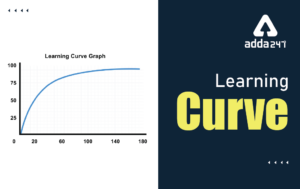Learning Curve - What is Learning Curve?...
•रस - परिभाषा, भे�...
•पठन कौशल- परिभा�...
•अलंकार - परिभाष�...
•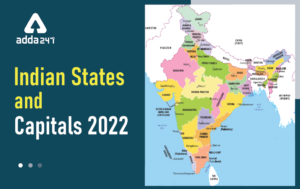Indian States and Capitals 2022: 28 Stat...
•New Education Policy(NEP) PDF - नई �...